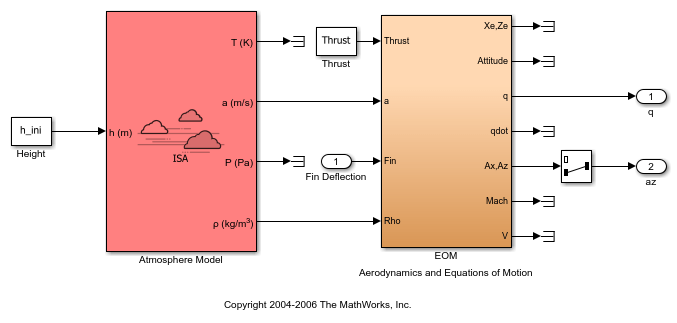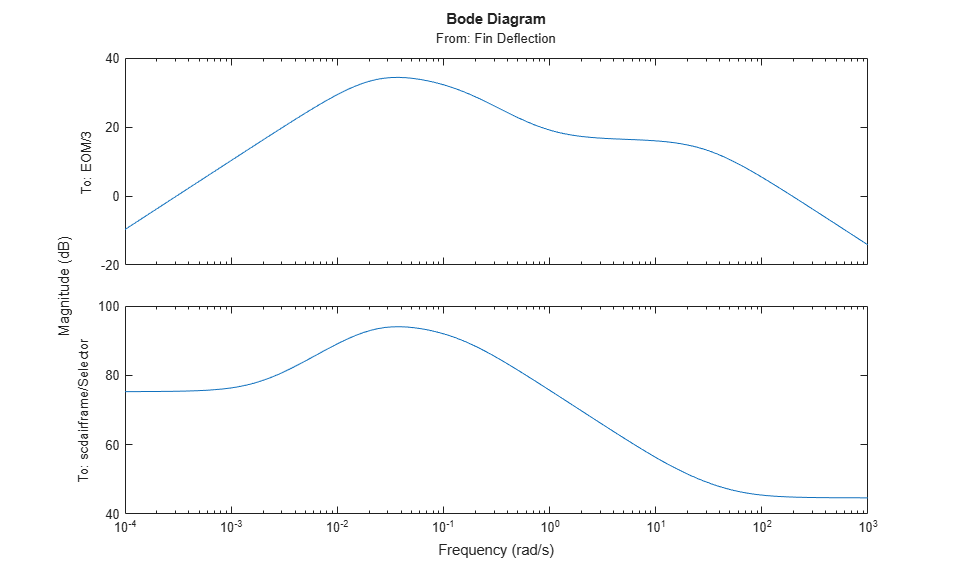# Trim and Linearize an Airframe

This example shows how to trim and linearize an airframe using Simulink® Control Design™ software.

The goal is to find the elevator deflection and the resulting trimmed body rate that generate a given angle of incidence when the airframe is traveling at a set speed.

Once you find the trim condition, you can compute a linear model for the dynamics of the states around the trim condition.

Fixed parameters:

• Angle of incidence (`Theta`)

• Body attitude (`U`)

• Position

• Elevator deflection (`w`)

• Body rate (`q`)

### Compute Operating Points

Open the model.

```mdl = 'scdairframe'; open_system(mdl) ```Create an operating point specification object for the model using the model initial conditions.

```opspec = operspec(mdl) ```
```opspec = Operating point specification for the Model scdairframe. (Time-Varying Components Evaluated at time t=0) States: ---------- x Known SteadyState Min Max dxMin dxMax ___________ ___________ ___________ ___________ ___________ ___________ ___________ (1.) scdairframe/EOM/ Equations of Motion (Body Axes)/Position 0 false true -Inf Inf -Inf Inf -3047.9999 false true -Inf Inf -Inf Inf (2.) scdairframe/EOM/ Equations of Motion (Body Axes)/Theta 0 false true -Inf Inf -Inf Inf (3.) scdairframe/EOM/ Equations of Motion (Body Axes)/U,w 984 false true -Inf Inf -Inf Inf 0 false true -Inf Inf -Inf Inf (4.) scdairframe/EOM/ Equations of Motion (Body Axes)/q 0 false true -Inf Inf -Inf Inf Inputs: ---------- u Known Min Max _____ _____ _____ _____ (1.) scdairframe/Fin Deflection 0 false -Inf Inf Outputs: ---------- y Known Min Max _____ _____ _____ _____ (1.) scdairframe/q 0 false -Inf Inf (2.) scdairframe/az 0 false -Inf Inf ```

Specify which states in the model are:

• Known at the operating point

• At steady state at the operating point

Specify that the `Position` states are known and not at steady state. For the state values, specified in `opspec.States(1).x`, use the default values from the model initial condition.

```opspec.States(1).Known = [1;1]; opspec.States(1).SteadyState = [0;0]; ```

Specify that the second state, which corresponds to the angle of incidence `Theta`, is known but not at steady state. As with the position states, use the default state value from the model initial condition.

```opspec.States(2).Known = 1; opspec.States(2).SteadyState = 0; ```

The third state specification includes the body axis angular rates `U` and `w`. Specify that both states are known at the operating point and that `w` is at steady state.

```opspec.States(3).Known = [1 1]; opspec.States(3).SteadyState = [0 1]; ```

Search for the operating point that meets these specifications.

```op = findop(mdl,opspec); ```
``` Operating point search report: --------------------------------- opreport = Operating point search report for the Model scdairframe. (Time-Varying Components Evaluated at time t=0) Operating point specifications were successfully met. States: ---------- Min x Max dxMin dx dxMax ___________ ___________ ___________ ___________ ___________ ___________ (1.) scdairframe/EOM/ Equations of Motion (Body Axes)/Position 0 0 0 -Inf 984 Inf -3047.9999 -3047.9999 -3047.9999 -Inf 0 Inf (2.) scdairframe/EOM/ Equations of Motion (Body Axes)/Theta 0 0 0 -Inf -0.0097235 Inf (3.) scdairframe/EOM/ Equations of Motion (Body Axes)/U,w 984 984 984 -Inf 22.6897 Inf 0 0 0 0 -1.4367e-11 0 (4.) scdairframe/EOM/ Equations of Motion (Body Axes)/q -Inf -0.0097235 Inf 0 6.3122e-16 0 Inputs: ---------- Min u Max _________ _________ _________ (1.) scdairframe/Fin Deflection -Inf 0.0014161 Inf Outputs: ---------- Min y Max __________ __________ __________ (1.) scdairframe/q -Inf -0.0097235 Inf (2.) scdairframe/az -Inf -0.24207 Inf ```

### Linearize Model

To linearize the model at the computed operating point, first specify the linearization input and output points.

```io(1) = linio('scdairframe/Fin Deflection',1,'input'); io(2) = linio('scdairframe/EOM',3,'output'); io(3) = linio('scdairframe/Selector',1,'output'); ```

Linearize the model at the operating point.

```sys = linearize(mdl,op,io); ```

Plot the Bode magnitude response for the linear model.

```bodemag(sys) bdclose('scdairframe') ```